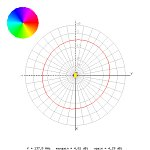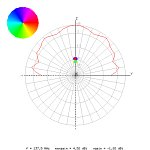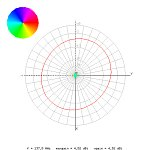Home
Antennas
QFH
Simulation

Language:
Español
Deutsch

Simulations
Dimensions
Groundplane

Related:
Simple helicoidal

## Simulation above a groundplane

 X-Axis Z-Axis HeightHeight above groundplane: 0.30 m (0.1375 wavelengths)Height above groundplane: 0.60 m (0.275 wavelengths)Height above groundplane: 0.90 m (0.4125 wavelengths)Height above groundplane: 1.20 m (0.55 wavelengths)Height above groundplane: 1.50 m (0.6875 wavelengths)Height above groundplane: 1.80 m (0.825 wavelengths)Height above groundplane: 2.10 m (0.9625 wavelengths)Height above groundplane: 2.40 m (1.1 wavelengths)Height above groundplane: 2.70 m (1.2375 wavelengths)Height above groundplane: 3.00 m (1.375 wavelengths)Height above groundplane: 3.30 m (1.5125 wavelengths)Height above groundplane: 3.60 m (1.65 wavelengths)Height above groundplane: inf m (0 wavelengths)

 (c) John Coppens ON6JC/LW3HAZ mail